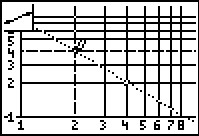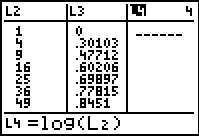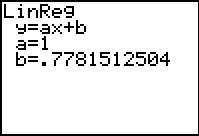### Mathematics lessons for IB® Diploma Programme

Applications and Interpretation | Numbers and Algebra## Properties of Logs

### Activity Overview

Students use a combination of algebra and graphing to discover the properties of logarithms.

## Key Steps## Step 1

In Problem 1, students investigate the power property of logarithms. Students use Lists to calculate the value of b = a2 for positive integral values of a.

Next, students define two new variables x = log a and y = log b and enter them into lists. Graphing this data in a scatter plot, students can use the LinReg command to determine that the graph of log a2 resembles the linear equation y = 2x. Rewriting this as a logarithmic equation, students obtain log a2 = 2 log a, revealing the Power Property of Logarithms.## Step 2

In Problem 2, students investigate the product property of logarithms. Students will again use Lists to do their investigation, this time to find the value of b = 6a, for given values of a.

Students graph the data in a scatter plot and find the y-intercept. Students should realize that this value is log 6. They can then rewrite the equation y = log 6 + x in terms of a. This will reveal the Product Property of Logarithms.## Step 3

Problem 3 gives students the opportunity to investigate the Quotient Property of logarithms. Students experiment with a logarithmic scale on both the x- and y-axes.